Journal DetailsFormat
Journal
eISSN
2083-831X
First Published
09 Nov 2012
Publication timeframe
4 times per year
Languages
EnglishOpen Access

# Influence of Permanent Deflections on The Vibrations of Bridge Spans in Operating Conditions

###### Accepted: 15 Dec 2021
Journal DetailsFormat
Journal
eISSN
2083-831X
First Published
09 Nov 2012
Publication timeframe
4 times per year
Languages
English

The paper presents the method and results of numerical simulations of the mutual dynamic interactions between vehicles and the bridge structure, which has defect in the form of excessive permanent deformations. The parametric analyses were carried out taking into account the following parameters: permanent deflections of spans, with a maximum value ranging from 0 to 150 mm, vehicle speed from 10 m/s (36 km/h) to 30 m/s (108 km/h), and two types of heavy vehicle suspension systems, each with very different properties. The presented analyses are based on the characteristics of real motorway bridge structure with permanent deflections of the multi-span main girders, constructed of steel beams and reinforced concrete slab. The proposed procedure of dynamic numerical analysis can also be useful in the assessment of the influence of permanent deflections on the interactions between vehicles and other types of bridge structures.

#### Keywords

Introduction

In engineering practice related to the maintenance and operation of road bridge infrastructure, we often deal with bridge spans that have permanent deflections. These types of defects may result from various causes such as uncontrolled displacements of scaffolding during the construction, rheological phenomena of structural concrete or overloading as a result of oversized vehicles or collisions. Changes in the grade line of a road surface that are caused by superfluous deflections of bridge spans often have a very significant influence on superstructure dynamic behaviour in operating conditions, mainly resulting from the mutual dynamic interaction between a bridge and vehicles. This issue is particularly important in relation to the health of existing, bridges located along motorways and expressways, on which there are many heavy vehicles and high traffic speeds.

Due to the occurrence of significant permanent deformations of the spans of few motorway bridges in Poland, an attempt was made to model and analyse the impact of this phenomenon on the structure performance in operational conditions. For this purpose, a numerical simulation analysis of the mutual dynamic interactions between vehicles and the considered bridge structure, which has defect in the form of excessive deformations, was carried out. Since the influence of many parameters on the dynamic response of the structure–vehicle system is random (e.g. [1, 2, 3, 4]), the parametric analyses were carried out taking into account the following factors:

– permanent deflections of spans, with a maximum value ranging from 0 to 150 mm,

– vehicle speed from 10 m/s (36 km/h) to 30 m/s (108 km/h) and

– two heavy vehicle suspension systems, each with very different properties.

During the analyses, main attention was paid to the values of the vertical displacements and accelerations of the vibrations of the bridge spans, as well as to the level of the increased dynamic loads induced by heavy vehicles on deformed superstructure.

Literature background

Both railway bridges and road bridges are particularly susceptible to dynamic effects in individual cases. It is widely known that the dynamic responses of bridges depend on the vehicle type, speed, type and roughness of the pavement, bridge type, etc. In previous years, a lot of attention in the literature had been dedicated to the analysis of the vibrations of the vehicle–bridge structure system, known in the literature as bridge–vehicle interaction problem. The conducted research concerned rail bridge structures (e.g. [4, 5, 6, 7, 8, 9, 10, 11, 12, 13]), with particular emphasis on the objects located along the high-speed railway lines (e.g. [10, 11, 12, 13]) as well as road bridges (e.g. [14, 15, 16, 17, 18, 19, 20, 21, 22, 23, 24, 25]).

In the case of studies related to the modelling and analysis of ‘road bridge–road vehicle’ dynamic interaction, four categories of research problems were usually investigated:

– vehicle–bridge dynamic interaction modelling methodology (e.g. [14, 15, 16, 17]),

– impact of condition of the road surface (e.g. road roughness, threshold irregularities, local surface defects) on the increase in dynamic wheel loads as well as on the overall dynamic response of the structure under live load (e.g. [18, 19, 20]),

– serviceability or condition assessment of bridge structures (e.g. [21, 22, 23]) and

– development of bridge weight-in-motion systems (e.g. [24-25]).

The purpose of this article is to assess the influence of permanent deflections of bridge spans on its dynamic response occurring during passages of heavy vehicles. According to the authors’ knowledge, the results of analyses related to the effect of permanent deflections of spans resulting in the corresponding deformation of a road surface on the overall dynamic behaviour of the structure under live load have not been published so far. Moreover, this effect is not included in the currently applicable recommendations for the assessment of existing bridge structures (e.g. [26, 27, 28, 29]; so far, in most of the countries, there are no standards for evaluating existing bridges). It requires an individual, time-history vibration analysis of the structure subjected to live loads.

The problem under consideration is quite a special case, very interesting from the scientific point of view and at the same time having practical significance for the management of existing road bridge infrastructure.

Structure of the analysed bridge

The considered bridge (Fig. 1) has two separate multi-span superstructures, each one located under a single motorway carriageway. The static diagram of the main girders of the bridge is a seven-span beam scheme, in which the continuity of the structure in support cross-section zones is assured using a thin reinforced concrete slab only (Fig. 2). The spans are designed as composite steel and concrete structures. Each of the spans consists of five steel I girders with a constant height of 2.00 m, which are casted with a 26-cm-thick reinforced concrete slab. The axial spacing of the girders is 2.50 m, and their theoretical span length is 41.00 m. The transverse slope of the slab along the deck’s width was obtained by varying the height of the slab slants above the plate girders from 5 to 27 cm. The basic dimensions of a single bridge span are shown in Fig. 3.

The composite structure of each span shows excessive permanent deflections of up to 10 cm, which are inconsistent with the design (Fig. 4). They are probably a result of the creep and shrinkage effect of the reinforced concrete slab, as well as of design and construction errors.

The girders are braced laterally with the X-type trusses located over the supports and every 6 m along the span.

The bridge spans are supported by steel roller bearings, hinged-sliding bearings and tangential non-sliding bearings, which are placed on the reinforced concrete pier caps. The abutments of the bridge are openwork structures embedded in the earthen embankments. The abutment’s foundation slabs are placed on bored piles that have a diameter of 1.50 m. The intermediate supports are frame structures. Each support is made of three piers with a diameter of 1.50 m and topped with a reinforced concrete pier cap with dimensions of 1.50 × 1.50 m.

During the inspection of the object, it was found that the spans showed excessive poorly damped vibrations, which were caused by typical traffic on the bridge. The spans’ vibrations were clearly perceptible and visible to the naked eye. The passage of even a single truck generated significant dynamic effects in terms of amplitudes of displacements and accelerations. In the case of the group of vehicles crossing a bridge (one after the other or side by side), a significant increase in vibration amplitudes was observed, which possibly was caused by the effect of synchronisation of vehicle vibrations with the vibrations of the structure.

The time needed to completely dampen the vibrations of the spans is relatively long and amounts to about 10–15 s. The value of the damping ratio ξ (%), which was determined on the basis of vibration measurements during inspection, ranges from 1% to 1.5%. Estimated damping ratio ξ (%) is slightly lower than for typical composite bridge superstructures (usually 1.5%–2.0%, e.g. [14, 15, 16, 17, 18, 19, 20, 21, 22, 23, 24, 25, 26, 27, 28]) and results from the simultaneous influence of the following factors: static system properties, features of the bearing system, type of connections of structural elements, etc. The relatively low damping value of the bridge spans is one of the factors influencing the excessive dynamic behaviour of the structure under operational loads.

Numerical model of the bridge

When a vehicle passes over a bridge span, there exists a mutually coupled dynamic system in which the interaction forces between the vehicle and the bridge are transmitted through the road surface. For many years, one of the most common theoretical approaches to examine the problem concerning bridge–vehicle interaction has been a method using two-dimensional models of both bridges and vehicles.[1,4,14,18] The two-dimensional system can provide good results (consistent with the results of experimental tests) for investigating the dynamic behaviour of the bridge spans under moving vehicles on uneven deck surface.

For the purpose of carrying out dynamic time-history vibration analyses using the Finite Element Method (FEM), the bridge superstructure was represented in the form of a (e1,p2) class model composed of one-dimensional elements located in a two-dimensional space. The model represents a single girder in the three consecutive most outer spans of the analysed structure (Figs 57). The single girder model should be considered as a virtually separated element from the superstructure, which can undergo vertical vibration only. On the basis of the preliminary analysis, the three-span model happened to be the most representative one and was assumed as a reference structure for further analysis.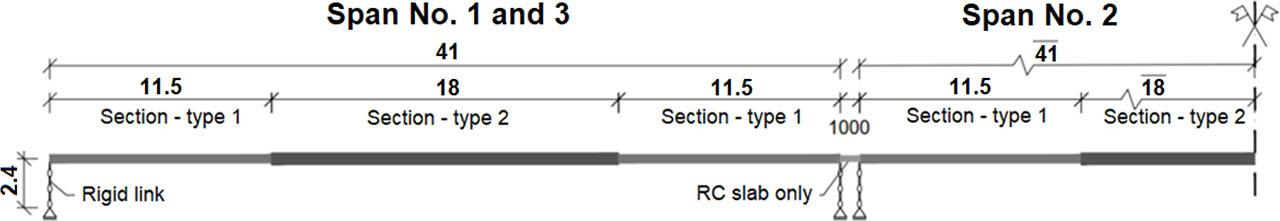Scheme of the spans’ geometry model – the three-span system was analysed; for the purpose of analyses, the spans were marked as 1, 2 and 3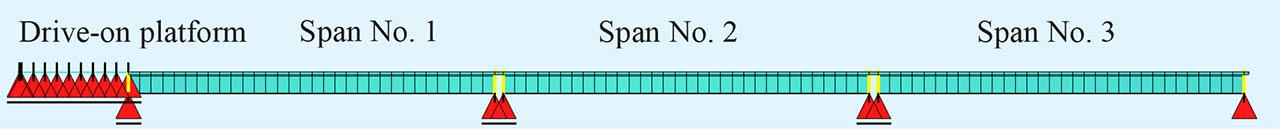Numerical model of the structure developed in the SOFiSTiK program – side view with spans marked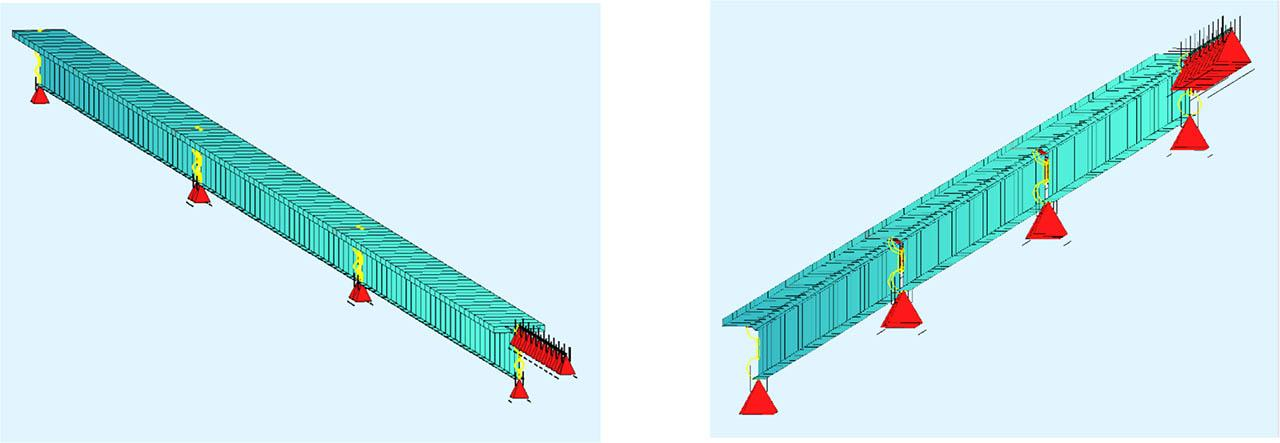Axonometric views of the numerical model of the structure developed in the SOFiSTiK program.

The bars in the model (the Navier–Bernoulli beam elements) were deprived of the possibility of rotation around the longitudinal axis along their length to prevent twisting of the elements. The cross sections of the FEM model bars are shown in Fig. 8. They represent the central girder (see Fig. 3), which is located directly under the right traffic lane and which withstands the greatest loads. The material parameters of the steel and reinforced concrete parts of the girders are given in Table 1.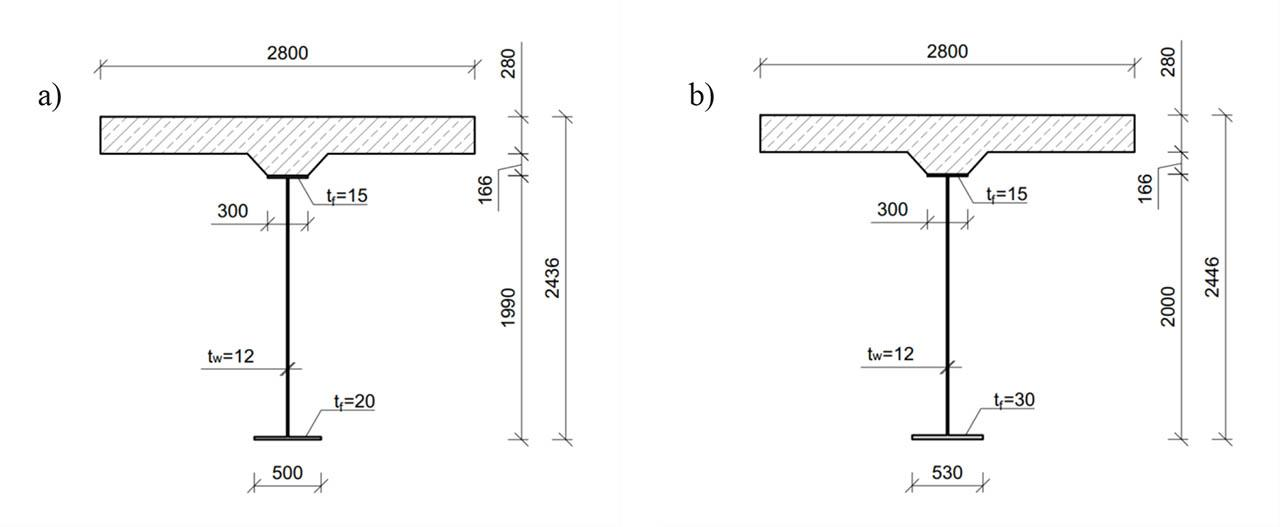Cross sections of the bar elements of the model of the superstructure: (a) cross section – type 1 (near the support), (b) cross section – type 2 (in the middle of the span)

Summary of material parameters of the steel and reinforced concrete parts of the bar elements of the FEM model.

Material characteristics Steel girder Reinforced concrete slab
Self-weight (volumetric weights additionally take into account the weight of the span's bracings and the spans’ equipment) γ 82.6 $(kNm3)$ \left({{{{\rm{kN}}} \over {{{\rm{m}}^3}}}} \right) 29.6 $(Nmm2)$ \left({{{\rm{N}} \over {{\rm{m}}{{\rm{m}}^2}}}} \right)
Young's modulus E 210,000.0 $(Nmm2)$ \left({{{\rm{N}} \over {{\rm{m}}{{\rm{m}}^2}}}} \right) 36000.0 $(Nmm2)$ \left({{{\rm{N}} \over {{\rm{m}}{{\rm{m}}^2}}}} \right)
Shear modulus G 80,769.0 $(Nmm2)$ \left({{{\rm{N}} \over {{\rm{m}}{{\rm{m}}^2}}}} \right) 15000.0 $(Nmm2)$ \left({{{\rm{N}} \over {{\rm{m}}{{\rm{m}}^2}}}} \right)
Poisson ratio μ 0.3 ( - ) 0.2 ( - )

Dynamic theoretical analyses were conducted with the use of two categories of models of the bridge span structure:

– a model without damage, that is, with ideal span geometry, in accordance with the designed grade line and

– a model with damage in the form of permanent deflections of the bridge spans, which were applied as equivalent geometric imperfections in relation to the original ideal geometry of the FEM model.

In the conducted simulations, permanent deflections with maximum values ranging from 0 to 150 mm were considered (these values correspond to the radius of curvature in the range from 1400 m to infinity). The deformed shape of the model representing permanent deflections was determined using the so-called primary load case (PLC) approach. In the first step of analysis, evenly distributed static load was applied to all spans, which allowed obtaining the desired deformed geometry of the system. Then, in the second step of analysis, dynamic time-history simulations of moving vehicle were performed according to the methodology described in Section 7, taking into account the deformed geometry of the system.

The applied modelling approach of bridge superstructure allows to achieve the assumed research goals, although it simplifies the vibration of the entire superstructure. In the next stages of research (not presented in this paper), it is also planned to conduct more advanced theoretical analyses using spatial models of structure and vehicle validated by the results of experimental tests.

The analysis of the dynamic behaviour of the bridge structure under the live loads not only requires the adoption of an effective numerical model of the structure, but also an effective mechanical model of a moving vehicle. In the presented simulation analyses, a typical five-axle truck with a total weight of 40 t was modelled as the live load (Fig. 9).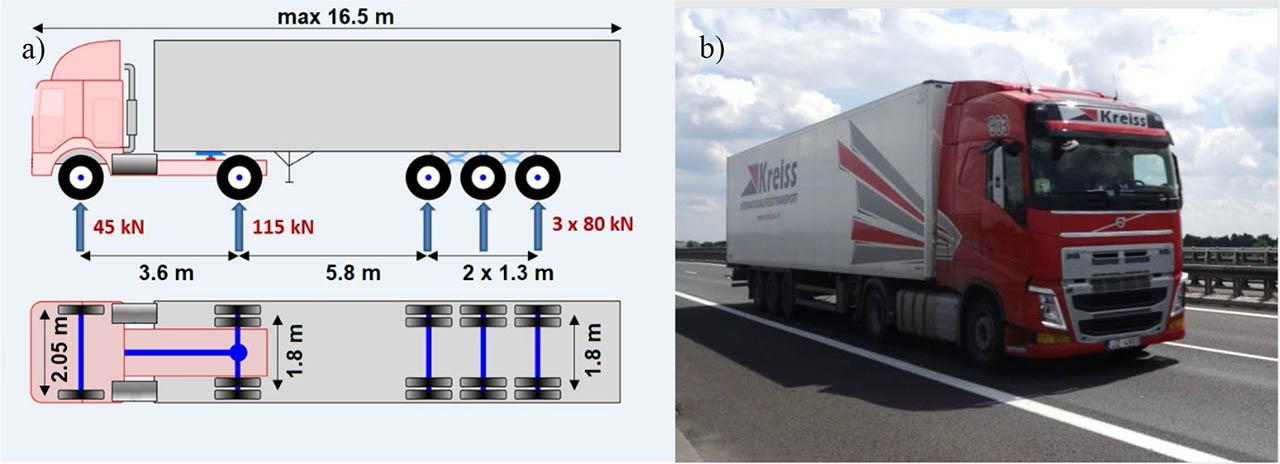Typical five-axle vehicle weighing 40 t: (a) wheelbase and loads of the vehicle’s axle, (b) general view of the vehicle passing through the considered facility

The actual suspension system of a truck with a semi-trailer (Fig. 9b) is characterised by the use of complex hydraulic and pneumatic systems, which, apart from damping the vibrations and levelling the effects of road unevenness, equalise the pressure on individual axles. In the presented analyses, a simplified suspension model was used, in which the vehicle load was reduced to five masses (M1–M2) supported by viscoelastic oscillators, individually described by stiffness (k1–k2) and damping (c1–c2) parameters (see Fig. 10). These mass oscillators correspond with individual suspension axles and are connected with each other by a bar of considerable stiffness, which has a hinge at the place of mass M2. It should be noted that in these types of models, certain issues, such as contact between the tire and the road surface, cannot be precisely taken into account. However, it is possible to properly select the parameters, so that the entire vehicle model is able to reproduce the specific dynamic characteristics of real vehicles.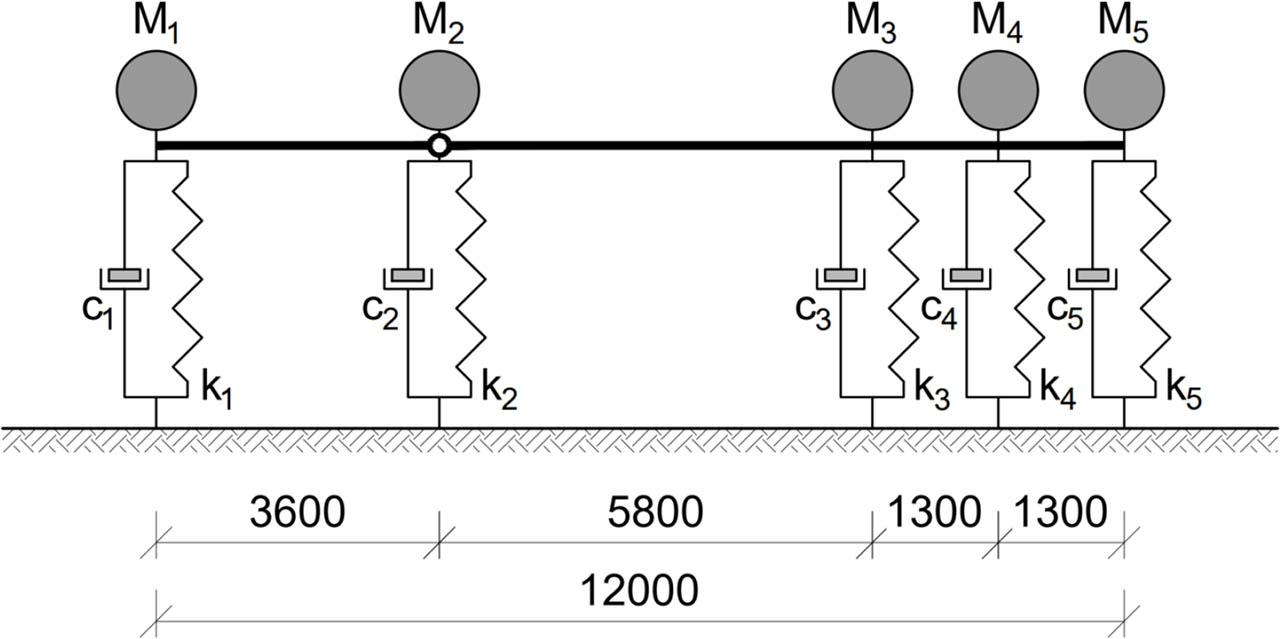The scheme of the suspension modelled as viscoelastic oscillators connected by a bar of considerable stiffness – modelling structure of vehicle.

The viscous damping ratio ξ (%) of the suspension system in new vehicles is most often in the range of 20%–35%. Values of the viscous damping coefficient in the range from 10% to 40% are also acceptable and do not indicate incorrect damping in the suspension system. A damping coefficient of 10% was assumed for further calculations.

Temporary (dynamic) values of the vertical components of loads from vehicle wheels acting on the surface change significantly while driving. In general, they depend on vehicle speed, vehicle suspension parameters, road surface condition, type of transported cargo or aerodynamic effects. Several dominant components can be distinguished in the frequency spectrum of the signal corresponding to vehicle vertical axle loads:

– components in the range of about 1.0–3.0 Hz related to the basic natural frequencies of the vehicle body vibrations; they result from the vibrations of the so-called sprung mass and

– a component, usually in the range of 8–10 Hz, which is related to the effect of suspension and wheel mass (the so-called unsprung mass) on the road surface.

Comprehensive modelling of the variability of vehicle wheel pressure on the surface of the bridge deck, which at the same time takes into account the interaction of the structure–vehicle systems, requires the adoption of very complex models that represent the dynamic characteristics of the vehicle (e.g. [7, 11, 12, 13]). In order to simplify the issue, in the presented analyses, two separate vehicles models were used:

– Model A – represents a vehicle with elastic suspension, in which the dominant component of the vehicle wheel loads in the frequency spectrum is equal to 2.3 Hz, and which is consistent with the first group of basic natural frequencies of the bridge spans model (see Table 3); the share of other components was omitted and

– Model B – represents a vehicle with rigid suspension, in which the dominant component of vehicle wheel loads in the frequency spectrum is equal to 7.5 Hz, and which is close to the second group of natural frequencies of the bridge spans model (see Table 3); the share of other components was omitted.

The basic parameters adopted in the developed live load models are presented in Table 2. The values of parameters were adopted based on the specialised technical literature (e.g. ).

Parameters of the numerical models of the considered vehicles.

Vehicle model Model A Model B
Basic natural frequencies 2.3 Hz 7.5 Hz
Number of generalised nodal displacements 5 5
Concentrated masses in the place of the axles M1=1.265 t M1=1.265 t
M2=2.415 t M2=2.415 t
M3–5=1.840 t M3–5=1.840 t
Suspension rigidity k1=263.9 kN/m k1=2806.0 kN/m
k2=503.8 kN/m k2=5357.0 kN/m
k3–5=383.9 kN/m k3–5==4082.0 kN/m
Suspension damping c1=3.654 kN/(m·s) c1==11.920 kN/(m·s)
c2=6.976 kN/(m·s) c2=22.750 kN/(m·s)
c3–5=5.315 kN/(m·s) c3–5=17.330 kN/(m·s)
Modal parameters of the structure

The dynamic parameters of the structure model were determined using the Simultaneous vector iteration method, which is available in SOFiSTiK software. The basic mode shapes of the analysed spans with their corresponding vibration frequencies are shown in Table 3. These features have a dominant effect on the vibrations of the structure under normal operation conditions. The first and the second groups of the natural frequencies of the bridge spans model are very similar to the typical frequencies of the vertical axle loads of heavy goods vehicles on the pavement (see Table 2). This is conducive to the occurrence of relatively large effects of dynamic interactions of vehicles and the structure.

Selected results of linear modal analysis using FEM.

 The first group of natural forms of vibrations The second group of natural forms of vibrations The third group of natural forms of vibrations

Moreover, a comprehensive dynamic analysis of bridge structures also requires the adoption of a vibration damping model as a function of time. It was assumed that the damping forces are linear, that is, directly proportional to the speed of the generalised nodal displacements of the FEM model, and are directed opposite to the direction of motion. This is called proportional damping and is also known as the equivalent viscous damping model. There are many different variants of equivalent viscous damping models, which are described, among others, in state-of-the-art monographs (e.g. [1, 2, 3]). In this work, the method of direct numerical integration of the system of equations (Newmark’s method) was used, and therefore, the proportional vibration damping model, called the Rayleigh damping model, was adopted in the calculations (e.g. [1, 2, 3]). In this model, the global damping matrix of structure C is described by the following formula: $C=αM+βK$ {\bf{C}} = \alpha {\bf{M}} + \beta {\bf{K}}

Coefficients of proportionality α and β in relation to the global matrix of the structure’s mass M and stiffness K were selected based on the natural frequencies of the structure model and the global damping coefficient ξ (%) of the real structure (determined based on the initial measurements of the bridge’s vibration). The α and β coefficients were calculated based on the following expressions: $α=ξ4πfafbfa+fb$ \alpha = \xi 4\pi {{{f_a}{f_b}} \over {{f_a} + {f_b}}} $β=ξ1π(fa+fb)$ \beta = \xi {1 \over {\pi ({f_a} + {f_b})}}

In the calculations, frequencies fa = 2.3 Hz and fb = 14.1 Hz were arbitrarily assumed. The value of fa is approximately consistent with the first group of the natural frequencies of the model, and the value of fb is close to the third group of natural frequencies of the model. In equation (2a, b), damping ratio ξ = 1 (%) was applied. The obtained values of the coefficients were equal to α = 0.249 and β = 0.0001936.

The Rayleigh damping model assumes a linear dependence of the damping ratio ξ on the vibration frequency f. A significant disadvantage of this model is the significantly higher values of the damping ratios ξ corresponding to the frequencies outside the fafb range. As a result, the global (considering the entire frequency range) damping ratio ξ related to free response vibrations of the model occurring after vehicle passage was approximately 1.2%–1.3% (for reference, see, e.g. Fig. 12; it can be noticed that it takes about 12 cycles of free vibration to reduce the amplitude of displacements of span 3 by half). This value is consistent with the one determined based on the initial measurements of the bridge’s vibration.

Methodology of the numerical analyses

A comprehensive picture of the theoretical dynamic response of bridge structures to live loads can be obtained using the time analysis of imposed vibrations. The formulation of the equations of motion, even for systems with several degrees of dynamic freedom, is a demanding task. Analytically, solutions can usually only be obtained for very basic problems. In practice, a necessary step in the dynamic analysis of complex systems is the development and analysis of the FEM numerical model.

In the analysis of the dynamic response of bridge structures to moving loads, methods of dynamic implicit analysis are usually used (e.g. [1, 2, 3]). In these methods, the values determined in step i + 1 depend on the value of the size in step i and in step i - 1. These methods are generally (non-linear problems) iterative methods. The Newmark method, which was used in this paper, is one of the implicit integration methods. Its basic theoretical assumptions are briefly discussed below.

In implicit integration methods, time is divided into discrete time periods ti∈{t1,…tn}, and then, the equation of motion of the model’s nodes is discretised, that is, the equation of motion of a dynamic system is transformed into the following form: $Mu¨(ti+1)+Cu˙(ti+1)+Ku(ti+1)=p(ti+1)$ {\bf{M\ddot u}}({t_{i + 1}}) + {\bf{C\dot u}}({t_{i + 1}}) + {\bf{Ku}}({t_{i + 1}}) = {\bf{p}}({t_{i + 1}}) where: ü (ti+1) – vector of the generalised vibration accelerations of nodes (in the direction of the degrees of freedom) in the time step i + 1, (ti+1) – vector of the generalised vibration velocities of nodes (in the direction of the degrees of freedom) in the time step i + 1, u(ti+1) – vector of the generalised displacements of nodes (in the direction of degrees of freedom) in the time step i + 1 and p(ti+1) – vector of equivalent nodal external loads, that is, equivalent loads of the system, which are applied to the nodes (in the direction of the degrees of freedom) in the i-th time step.

In the subsequent time steps i+1 of the analysis, an approximate solution of the system of equation (1) as a function of the discrete time variable is sought based on the quantities calculated for the previous time step ti, while at the same time taking into account the initial conditions. The variability of the velocity of displacements and accelerations of vibrations, in particular sub-intervals [ti, ti+1], is approximated, where ti+1= tit.

The length of integration step Δt is a key parameter that influences the accuracy of the obtained solution. The value of this parameter should be a fraction of the period of natural frequencies (with higher frequencies). In the presented analyses, the time step was assumed as Δt = 0.001 s to meet the condition Δt< 0.1/fmax.

The values of the velocity and the vibration displacements at the end of the time step are calculated from the following formulas based on the values (ti) and u(ti), which are known at the beginning of the time step: $u˙(ti+1)=u˙(ti)+∫0Δtu¨(τ)dτ$ {\bf{\dot u}}({t_{i + 1}}) = {\bf{\dot u}}({t_i}) + \int_0^{\Delta t} {{\bf{\ddot u}}(\tau)d\tau} $u(ti+1)=u(ti)+∫0Δtu˙(τ)dτ$ {\bf{u}}({t_{i + 1}}) = {\bf{u}}({t_i}) + \int_0^{\Delta t} {{\bf{\dot u}}(\tau)d\tau} where τ is a local time variable in a particular time subinterval [ti, ti+1].

Solving equations (4) and (5) requires the determination of the arbitrary nature of changes in acceleration ü(ti) during a single time step. The analyses assumed a constant value of acceleration in the subintervals [ti, ti+1]. This is called the average acceleration method, in which the changes in vibration accelerations during the time step can be formulated as follows: $u¨(τ)=u¨(ti)+τΔt[u¨(ti+1)−u¨(ti)]$ {\bf{\ddot u}}(\tau) = {\bf{\ddot u}}({t_i}) + {\tau \over {\Delta t}}[{\bf{\ddot u}}({t_{i + 1}}) - {\bf{\ddot u}}({t_i})]

Alternatively, a linear change of acceleration over a time step can be assumed (linear acceleration method). The nature of changes in physical quantities during a single time step, depending on an arbitrarily adopted change in vibration accelerations, is shown in Fig. 11.

In the Newmark method, for the velocity and displacement at the end of the time interval Δt, the following conditions must be met: $u˙(ti+1)=u˙(ti)+Δt[(1−δ)u¨(ti)+δu¨(ti+1)]$ {\bf{\dot u}}({t_{i + 1}}) = {\bf{\dot u}}({t_i}) + \Delta t[(1 - \delta){\bf{\ddot u}}({t_i}) + \delta {\bf{\ddot u}}({t_{i + 1}})] $u(ti+1)=u(ti)+Δtu˙(ti)Δt2[(1/2−β)u¨(ti)+βu¨(ti+1)]$ \matrix{{{\bf{u}}({t_{i + 1}}) = {\bf{u}}({t_i}) + \Delta t{\bf{\dot u}}({t_i})} \cr {\Delta {t^2}[(1/2 - \beta){\bf{\ddot u}}({t_i}) + \beta {\bf{\ddot u}}({t_{i + 1}})]} \cr}

In the presented analysis, the parameter values that are used in the standard Newmark method were adopted, that is, δ=0.5, β=0.25(0.5+δ)2.

Parametric analysis results

A comprehensive parametric analysis of the dynamic interaction of the developed models of a heavy truck and the considered bridge structure with damage in the form of excessive deformation was carried out. In subsequent simulations of the vehicle driving over the bridge, the impact of changing one simulation factor (with the set values of other parameters) on the dynamic response of bridge model was investigated.

A total of 100 simulations were performed. During the analyses, main attention was paid to the values of the vertical displacements and accelerations of vibrations of the bridge spans, which were generated by heavy vehicles in the main girders as a function of the intensity of permanent deflections of the superstructure. The selected results of the calculations simulating passages of a five-axle vehicle weighing 40 t are shown in Figs 1217:

Figs 1214 show the changes in the vertical displacements of the cross section located in the middle of span 3 of the structure model (see the numbering of the spans of the model in Fig. 6) and

Figs 1517 show changes in the acceleration of vertical vibrations in the middle of span 3 of the structure model.

The changes in the physical quantities are presented as a function of various parameters:

– values of permanent deflections of spans: 0, 50, 100 and 150 mm,

– vehicle suspension stiffness: Model A – elastic suspension, Model B – rigid suspension and

– vehicle speed, during which the dynamic effects in the structure were usually the highest: 23, 26.8 and 30 m/s.

Additionally, the results of the dynamic displacements of the considered cross sections of the spans were compared with the static deflections generated by the same vehicle.

Changes in the calculated values as a function of time are presented in Figs 1217. In this cross section, the dynamic effects generated in the structure were significantly greater when compared to the values generated in the remaining spans. The presented results include bridge model vibrations induced by the direct impact of the vehicle, as well as free vibrations that result from the vehicle leaving the structure.

It is also worth noting the rumble effect visible in the free vibrations’ response (see Figs 1217), which is caused by mutual amplification of vibrations of adjacent spans. This effect results from closely spaced modes of the superstructure structural system (see Table 3) and it may be one of the key factors influencing the so-called dynamic sensitivity of the analysed structure.

The extreme values of the calculated physical quantities for all the numerical simulations (for all passages of vehicles) are shown in Figs 18 and 19:

Fig. 18 shows the maximum values of the deflections of the cross section located in the middle of spans 1–3 (see the numbering of the model spans in Fig. 18).

Fig. 19 shows the extreme values of the vertical vibration accelerations of the cross section located in the middle of spans 1–3.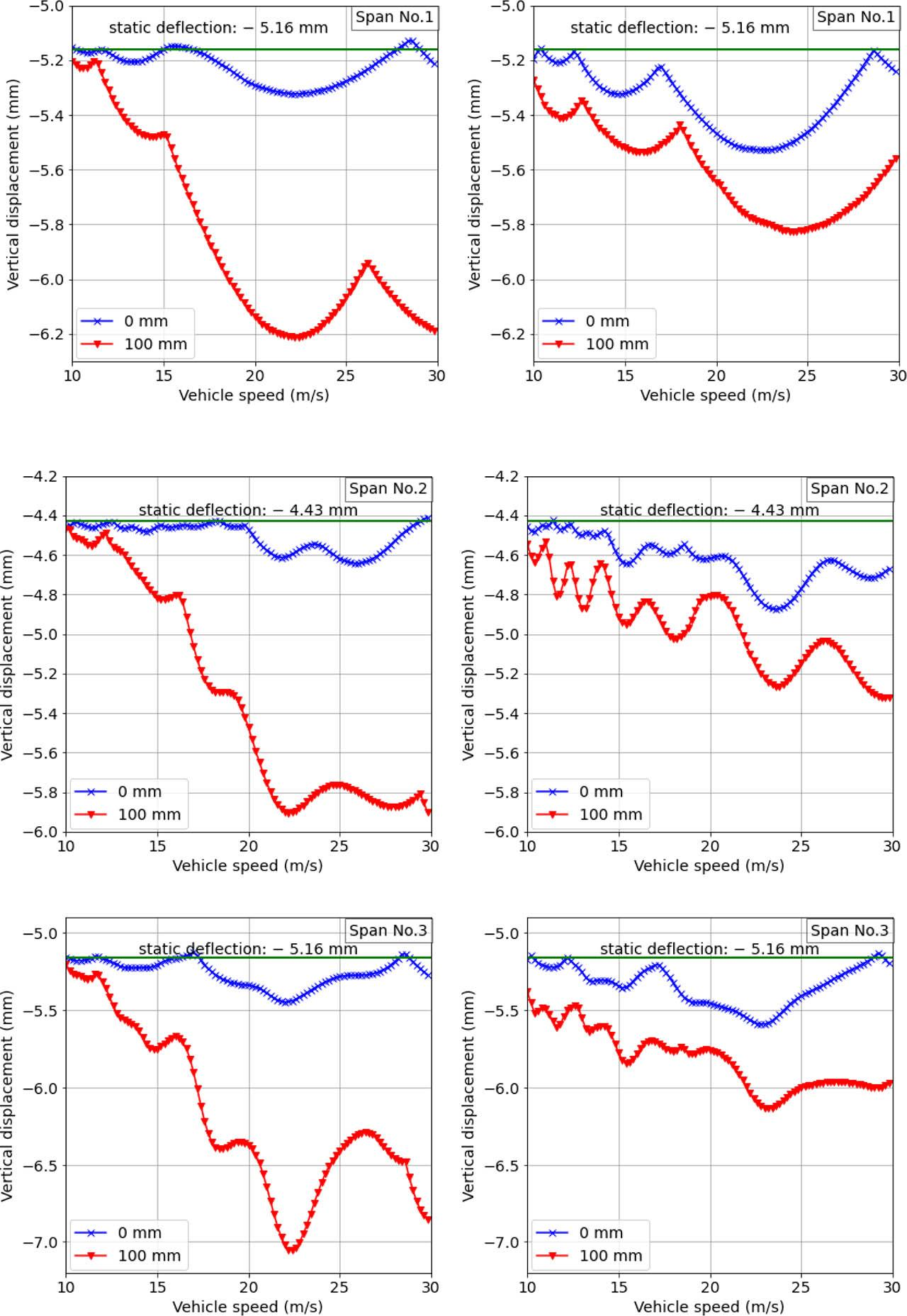The maximum deflections of the cross section located in the middle of spans 1–3 as a function of the vehicle speed and the value of permanent deflections (0 and 100 mm, respectively): Model A (elastic suspension; left), Model B (rigid suspension; right).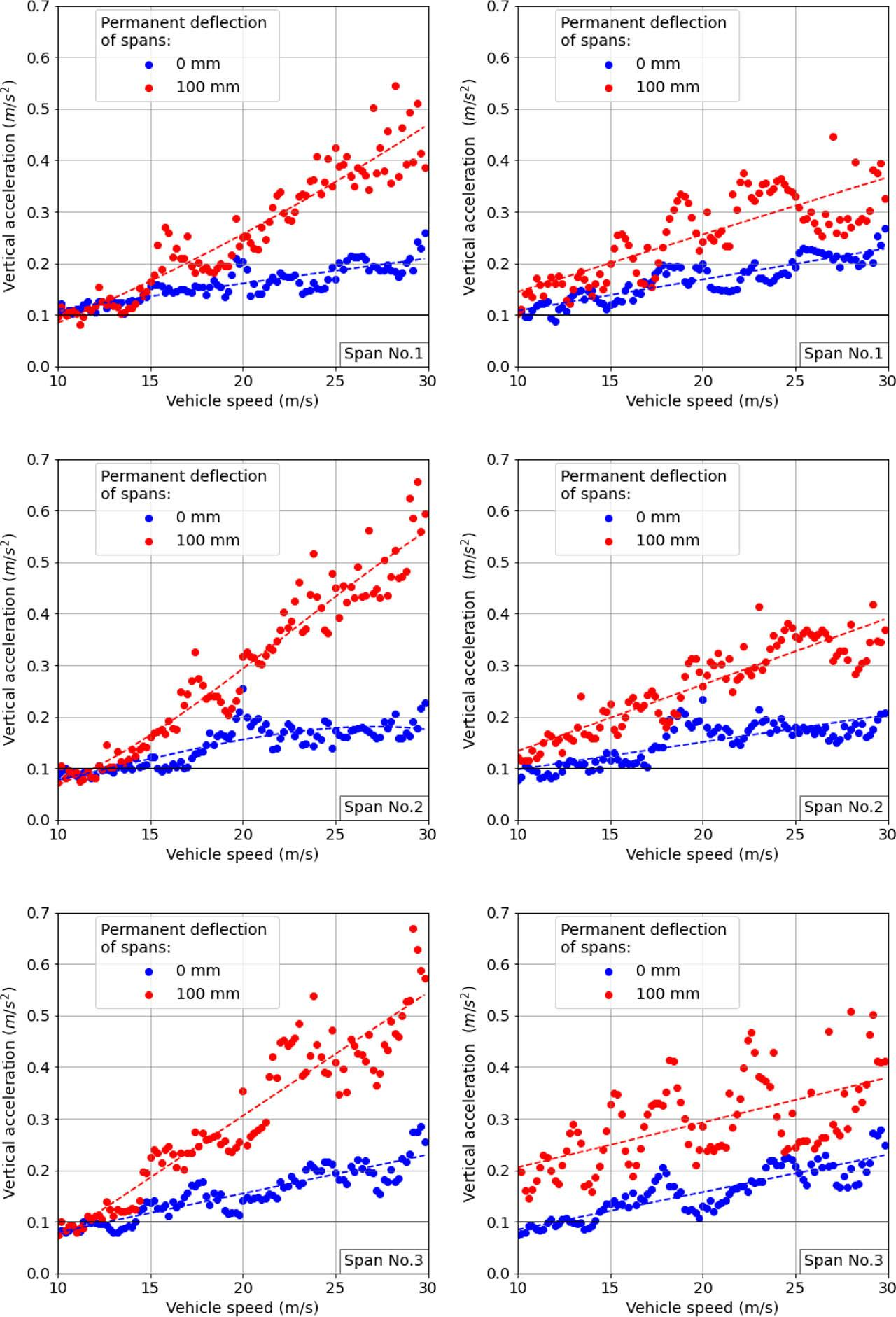The maximum vibration accelerations of the cross section located in the middle of spans 1–3 as a function of the vehicle speed and the value of permanent deflections (0 and 100 mm, respectively): Model A (elastic suspension; left), Model B (rigid suspension; right).

The results are presented as a function of the vehicle speed (ranging from 10 to 30 m/s; the ‘speed-step’ was equal to 0.2 m/s) for the structure without permanent deflections (0 mm) and for the intensity of the permanent deflections of 100 mm in each span.

Changes in the dynamic amplification factor (DAF) imposed by the passage of the vehicle as a function of the value of the permanent deflections of the spans are shown in Fig. 20. DAF parameters, which depict increase in the global dynamic effects in the structure, were determined as the ratio of dynamic to static displacements in the cross sections located in the middle of spans 1–3. The results were presented as a function of the value of the permanent deflections of the spans (0–150 mm) with regards to the fixed value of the vehicle speed (equal to 23 m/s) and different stiffnesses of the vehicle suspension (Model A – elastic suspension, Model B – rigid suspension).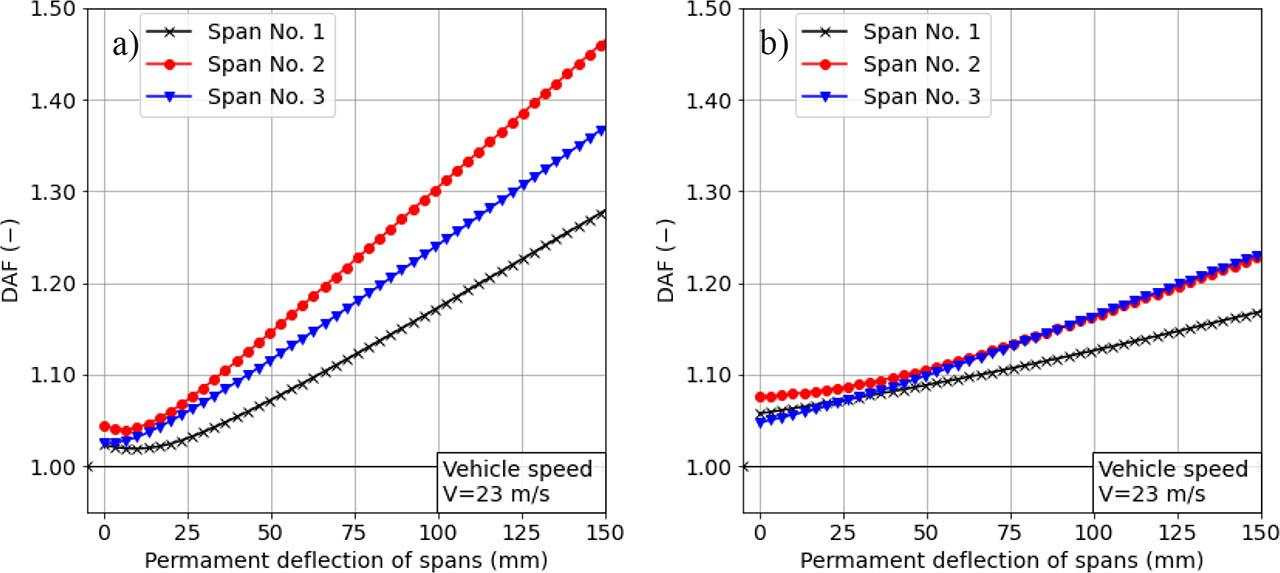Changes in the DAF imposed by the passage of the vehicle (which were read in the cross sections located in the middle of spans 1–3) as a function of the value of the permanent deflections of the spans and the determined value of the vehicle speed equal to 23 m/s: (a) Model A (elastic suspension), (b) Model B (rigid suspension).
Analysis of the results and summary

Based on the analysis of the results of the numerical simulations, the following conclusions can be drawn:

– The following factors have a significant influence on the dynamic response of a bridge structure in the context of both displacements and vibration accelerations:

– the speed of the vehicle passage through the bridge,

– range of the permanent deflections of the bridge superstructure and

– stiffness of the vehicle suspension (the effect of different suspension damping values was not analysed).

An increase in the values of the above-mentioned parameters results in a significant increase in the dynamic effects in the bridge spans.

– The permanent deflections of the spans have the greatest impact on increase in the dynamic effects in the analysed bridge. Permanent deformations of the spans’ grade line change the trajectory of vehicle movement, which causes forced vibrations of the vehicle itself, as well as their interaction with the vibrations of the structure. Finally, they usually result in increased vibrations of the entire vehicle–structure system and increased values of dynamic loads.

– Extreme dynamic effects in the considered structure (with regards to deflections and accelerations of vibrations) were most often obtained in the cross section located in the middle of span 3 during the passage of vehicles at a speed of about 23 m/s. For example, for the permanent deflection of the spans, which is equal to 100 mm:

– the calculated value of the maximum dynamic deflection in span number 3 is 30% greater than the maximum static deflection and

– the value of the maximum vibration acceleration in span number 3 is almost 3 times greater than the maximum vibration acceleration when the same vehicle passes at the same speed, but along a structure with a perfect geometry.

– Based on the results of the analyses, it can be concluded that the speed of the considered type of truck, which is equal to about 23 m/s (82.8 km/h), is the so-called critical speed. In such cases, the amplitudes of physical quantities that arise in the structure of the bridge spans have significant values.

– The increase in the global dynamic effects in the structure, which are generated by vehicle traffic, is approximately directly proportional to the value of the permanent deflections (Fig. 20). This phenomenon is related to the formation of forces (increased values of interactions) caused by the mass inertia of vehicles and the mutual interaction of the vehicle–structure subsystems.

In addition, it should be taken into account that the increased dynamic effects cause increased stresses (the difference between the maximum and minimum values at the structural notch), which are associated with the vibrations of the span’s elements – in particular, the main girders. This can significantly reduce the fatigue life of the structure of the spans.

The proposed procedure of dynamic numerical simulations can also be useful in the analysis of other types of bridge structures with permanent deflections. Further stages of research will involve the validation of the obtained results of the analyses based on the results of monitoring and dynamic experimental tests carried out during normal operation of the considered bridge. Moreover, it is planned to conduct analogous simulation analyses using a comprehensive spatial FEM model, which would take into account all the elements of the bridge’s spans.

#### Selected results of linear modal analysis using FEM.

 The first group of natural forms of vibrations The second group of natural forms of vibrations The third group of natural forms of vibrations

#### Parameters of the numerical models of the considered vehicles.

Vehicle model Model A Model B
Basic natural frequencies 2.3 Hz 7.5 Hz
Number of generalised nodal displacements 5 5
Concentrated masses in the place of the axles M1=1.265 t M1=1.265 t
M2=2.415 t M2=2.415 t
M3–5=1.840 t M3–5=1.840 t
Suspension rigidity k1=263.9 kN/m k1=2806.0 kN/m
k2=503.8 kN/m k2=5357.0 kN/m
k3–5=383.9 kN/m k3–5==4082.0 kN/m
Suspension damping c1=3.654 kN/(m·s) c1==11.920 kN/(m·s)
c2=6.976 kN/(m·s) c2=22.750 kN/(m·s)
c3–5=5.315 kN/(m·s) c3–5=17.330 kN/(m·s)

#### Summary of material parameters of the steel and reinforced concrete parts of the bar elements of the FEM model.

Material characteristics Steel girder Reinforced concrete slab
Self-weight (volumetric weights additionally take into account the weight of the span's bracings and the spans’ equipment) γ 82.6 (kNm3) \left({{{{\rm{kN}}} \over {{{\rm{m}}^3}}}} \right) 29.6 (Nmm2) \left({{{\rm{N}} \over {{\rm{m}}{{\rm{m}}^2}}}} \right)
Young's modulus E 210,000.0 (Nmm2) \left({{{\rm{N}} \over {{\rm{m}}{{\rm{m}}^2}}}} \right) 36000.0 (Nmm2) \left({{{\rm{N}} \over {{\rm{m}}{{\rm{m}}^2}}}} \right)
Shear modulus G 80,769.0 (Nmm2) \left({{{\rm{N}} \over {{\rm{m}}{{\rm{m}}^2}}}} \right) 15000.0 (Nmm2) \left({{{\rm{N}} \over {{\rm{m}}{{\rm{m}}^2}}}} \right)
Poisson ratio μ 0.3 ( - ) 0.2 ( - )

Fryba, L. (1999). Vibrations of Solids and Structure Under Moving Loads, 3-rd.ed., London: Thomas Telford. FrybaL. 1999 Vibrations of Solids and Structure Under Moving Loads 3-rd.ed. London Thomas Telford 10.1680/vosasuml.35393 Search in Google Scholar

Craig R.R. Jr., Kudrila A.J., Fundamentals of structural dynamics, John Wiley & Sons, Inc., Hoboken, New Jersey, 2006. CraigR.R.Jr. KudrilaA.J. Fundamentals of structural dynamics John Wiley & Sons, Inc. Hoboken, New Jersey 2006 Search in Google Scholar

Paultre P., Dynamics of Structures, ISTE Ltd and John Wiley & Sons, Inc., 2020. PaultreP. Dynamics of Structures ISTE Ltd and John Wiley & Sons, Inc. 2020 Search in Google Scholar

Klasztorny, Dynamics of Beam Bridges Loaded by High-Speed Trains, WNT Press, Warsaw, 2005, (in Polish). Klasztorny Dynamics of Beam Bridges Loaded by High-Speed Trains WNT Press Warsaw 2005 (in Polish). Search in Google Scholar

Q.-L. Zhang, A. Vrouwenvelder, and J. Wardenier, “Numerical simulation of train – bridge interactive dynamics”, Comput. Struct. 79, 1059–1075 (2001). ZhangQ.-L. VrouwenvelderA. WardenierJ. “Numerical simulation of train – bridge interactive dynamics” Comput. Struct. 79 1059 1075 2001 10.1016/S0045-7949(00)00181-4 Search in Google Scholar

Wanming Zhai, Zhaoling Han, Zhaowei Chen, Liang Ling & Shengyang Zhu (2019) Train–track–bridge dynamic interaction: a state-of-the-art review, Vehicle System Dynamics, 57:7, 984–1027. ZhaiWanming HanZhaoling ChenZhaowei LingLiang ZhuShengyang 2019 Train–track–bridge dynamic interaction: a state-of-the-art review Vehicle System Dynamics 57 7 984 1027 10.1080/00423114.2019.1605085 Search in Google Scholar

Szafrański M., Impact of rolling stock on railway bridges at variable traffic parameters, doctoral dissertation, Gdansk University of Technology, Gdansk 2014, (in Polish). SzafrańskiM. Impact of rolling stock on railway bridges at variable traffic parameters doctoral dissertation, Gdansk University of Technology Gdansk 2014 (in Polish). Search in Google Scholar

Szafrański M., A dynamic vehicle-bridge model based on the modal identification results of an existing EN57 train and bridge spans with non-ballasted tracks, Mechanical Systems and Signal Processing, Vol. 146, No 107039, 2021. SzafrańskiM. A dynamic vehicle-bridge model based on the modal identification results of an existing EN57 train and bridge spans with non-ballasted tracks Mechanical Systems and Signal Processing 146 107039 2021 10.1016/j.ymssp.2020.107039 Search in Google Scholar

Szafrański, Dynamics of the small-span railway bridge under moving loads, MATEC Web of Conferences, Vol. 262, No 10014, 2019. Szafrański Dynamics of the small-span railway bridge under moving loads MATEC Web of Conferences 262 10014 2019 10.1051/matecconf/201926210014 Search in Google Scholar

M.-K. Song, H.-C. Noh, and C.-K. Choi, “A new three dimensional finite element analysis model of high-speed train – bridge interactions”, Engineering Structures 25, 1611–1626 (2003). SongM.-K. NohH.-C. ChoiC.-K. “A new three dimensional finite element analysis model of high-speed train – bridge interactions” Engineering Structures 25 1611 1626 2003 10.1016/S0141-0296(03)00133-0 Search in Google Scholar

Y B Yang, J D Yau, Y S Wu, Vehicle–Bridge Interaction Dynamics With Applications to High-Speed Railways, World Scientific, July 2004, https://doi.org/10.1142/5541 YangY B YauJ D WuY S Vehicle–Bridge Interaction Dynamics With Applications to High-Speed Railways World Scientific July 2004 https://doi.org/10.1142/5541 10.1142/5541 Search in Google Scholar

M. Podworna and M. Klasztorny, “Vertical vibrations of composite bridge/track structure/high-speed train system. Part 1: Bull. Pol. Ac.: Tech. 62(2) 2014 319 62 (1), 165–180 (2014). PodwornaM. KlasztornyM. “Vertical vibrations of composite bridge/track structure/high-speed train system. Part 1: Bull. Pol. Ac.: Tech. 62 2 2014 319 62 (1), 165–180 (2014). Search in Google Scholar

M. Podworna and M. Klasztorny, “Vertical vibrations of composite bridge/track structure/high-speed train system. Part 2: Physical and mathematical modelling”, Bull. Pol. Ac.: Tech. 62 (1), 181–196 (2014). PodwornaM. KlasztornyM. “Vertical vibrations of composite bridge/track structure/high-speed train system. Part 2: Physical and mathematical modelling” Bull. Pol. Ac.: Tech. 62 1 181 196 2014 10.2478/bpasts-2014-0019 Search in Google Scholar

Cantieni R. Dynamic behavior of highway bridges under the passage of heavy vehicles. EMPA Report No. 220, 1992. CantieniR. Dynamic behavior of highway bridges under the passage of heavy vehicles EMPA Report No. 220, 1992 Search in Google Scholar

Green MF, Cebon D., Dynamic interaction between heavy vehicles and highway bridges, Computers and Structures, Vol 62, No 2, pp 253–264, 1997. GreenMF CebonD Dynamic interaction between heavy vehicles and highway bridges Computers and Structures 62 2 253 264 1997 10.1016/S0045-7949(96)00198-8 Search in Google Scholar

Kwasniewski L., Lib H., Wekezer J., Malachowski J., Finite element analysis of vehicle–bridge interaction, Finite Elements in Analysis and Design 42, 950–959, 2006. KwasniewskiL. LibH. WekezerJ. MalachowskiJ. Finite element analysis of vehicle–bridge interaction Finite Elements in Analysis and Design 42 950 959 2006 10.1016/j.finel.2006.01.014 Search in Google Scholar

Zhang Y., Zhao H., Lie S.T., A nonlinear multi-spring tire model for dynamic analysis of vehicle-bridge interaction system considering separation and road roughness, Journal of Sound and Vibration 436, 112–137, 2018. ZhangY. ZhaoH. LieS.T. A nonlinear multi-spring tire model for dynamic analysis of vehicle-bridge interaction system considering separation and road roughness Journal of Sound and Vibration 436 112 137 2018 10.1016/j.jsv.2018.08.039 Search in Google Scholar

Kim C.W., Kawatani M., Kim K.B., Three-dimensional dynamic analysis for bridge–vehicle interaction with roadway roughness, Computers and Structures 83, 1627–1645, 2005. KimC.W. KawataniM. KimK.B. Three-dimensional dynamic analysis for bridge–vehicle interaction with roadway roughness Computers and Structures 83 1627 1645 2005 10.1016/j.compstruc.2004.12.004 Search in Google Scholar

Oliva J., Goicolea J.M., Antolín P., Astiz M.A., Relevance of a complete road surface description in vehicle–bridge interaction dynamics, Engineering Structures 56, 466–476, 2013. OlivaJ. GoicoleaJ.M. AntolínP. AstizM.A. Relevance of a complete road surface description in vehicle–bridge interaction dynamics Engineering Structures 56 466 476 2013 10.1016/j.engstruct.2013.05.029 Search in Google Scholar

Dinga L., Hao H., Zhu X., Evaluation of dynamic vehicle axle loads on bridges with different surface conditions, Journal of Sound and Vibration 323, 826–848, 2009. DingaL. HaoH. ZhuX. Evaluation of dynamic vehicle axle loads on bridges with different surface conditions Journal of Sound and Vibration 323 826 848 2009 10.1016/j.jsv.2009.01.051 Search in Google Scholar

Caia C.S., Shia X.M., Araujoa M., Chenb S.R., Effect of approach span condition on vehicle-induced dynamic response of slab-on-girder road bridges, Engineering Structures 29, 3210–3226, 2007. CaiaC.S. ShiaX.M. AraujoaM. ChenbS.R. Effect of approach span condition on vehicle-induced dynamic response of slab-on-girder road bridges Engineering Structures 29 3210 3226 2007 10.1016/j.engstruct.2007.10.004 Search in Google Scholar

Li H., Wekezer J.W., Kwasniewski L., Dynamic Response of a Highway Bridge Subjected to Moving Vehicles, Journal of Bridge Engineering 13(5), 2008. LiH. WekezerJ.W. KwasniewskiL. Dynamic Response of a Highway Bridge Subjected to Moving Vehicles Journal of Bridge Engineering 13 5 2008 10.1061/(ASCE)1084-0702(2008)13:5(439) Search in Google Scholar

Camara A., Nguyen K., Ruiz-Teran A.M., Stafford P.J., Serviceability limit state of vibrations in under-deck cable-stayed bridges accounting for vehicle-structure interaction, Engineering Structures 61, 61–72, 2014. CamaraA. NguyenK. Ruiz-TeranA.M. StaffordP.J. Serviceability limit state of vibrations in under-deck cable-stayed bridges accounting for vehicle-structure interaction Engineering Structures 61 61 72 2014 10.1016/j.engstruct.2013.12.030 Search in Google Scholar

Sujon M., Dai F., Application of weigh-in-motion technologies for pavement and bridge response monitoring: State-of-the-art review, Automation in Construction 130, 103844, 2021. SujonM. DaiF. Application of weigh-in-motion technologies for pavement and bridge response monitoring: State-of-the-art review Automation in Construction 130 103844 2021 10.1016/j.autcon.2021.103844 Search in Google Scholar

Lansdell A., Song W., Dixon B., Development and testing of a bridge weigh-in-motion method considering nonconstant vehicle speed, Engineering Structures 152, 709–726, 2017. LansdellA. SongW. DixonB. Development and testing of a bridge weigh-in-motion method considering nonconstant vehicle speed Engineering Structures 152 709 726 2017 10.1016/j.engstruct.2017.09.044 Search in Google Scholar

Guideline for modelling of deterioration in bridges, Deliverable D11, Final Report. Bridge Management in Europe – BRIME. Research Project, 4th Framework Programme, 1999 r. Guideline for modelling of deterioration in bridges, Deliverable D11, Final Report Bridge Management in Europe – BRIME. Research Project, 4th Framework Programme, 1999 r. Search in Google Scholar

Guideline for Load and Resistance Assessment of Railway Bridges. Final Report, Integrated Research Project “Sustainable Bridges”, European Commission, 6th Framework Programme, 2007 r. Guideline for Load and Resistance Assessment of Railway Bridges Final Report, Integrated Research Project “Sustainable Bridges”, European Commission, 6th Framework Programme, 2007 r Search in Google Scholar

Guideline for the Assessment of Existing Structures. Final Report, Research Project “Structural Assessment Monitoring and Control – SAMCO”, 2006. Guideline for the Assessment of Existing Structures Final Report, Research Project “Structural Assessment Monitoring and Control – SAMCO” 2006 Search in Google Scholar

Quality specifications for roadway bridges, standardization at a European level. COST Action TU1406, 2015–2019 r., Project Reports: https://www.tu1406.eu/ Quality specifications for roadway bridges, standardization at a European level COST Action TU1406, 2015–2019 r., Project Reports: https://www.tu1406.eu/ Search in Google Scholar

Burnos P., Weighing vehicles in motion, Part 2: Types and Characteristics of Weigh In Motion (WIM) Systems, „Drogownictwo” 7–8, 2014, (in Polish). BurnosP. Weighing vehicles in motion, Part 2: Types and Characteristics of Weigh In Motion (WIM) Systems „Drogownictwo” 7 8 2014 (in Polish). Search in Google Scholar

Prochowski L., Żuchowski A., Trucks and buses, Wydawnictwa komunikacji i Łączności, 2016, (in Polish). ProchowskiL. ŻuchowskiA. Trucks and buses, Wydawnictwa komunikacji i Łączności 2016 (in Polish). Search in Google Scholar

Rutishauser H., Simultaneous Iteration Method for Symmetric Matrices, Handbook for Automatic Computation, 284–302, 1970 RutishauserH. Simultaneous Iteration Method for Symmetric Matrices Handbook for Automatic Computation 284 302 1970 10.1007/978-3-642-86940-2_20 Search in Google Scholar

SOFISTIK software manual: ASE General Static Analysis module. SOFiSTiK AG company, 2020. SOFISTIK software manual: ASE General Static Analysis module SOFiSTiK AG company 2020 Search in Google Scholar

Newmark N.M., A method of computation for structural dynamics, Journal of Engineering Mechanics, ASCE 1959, 85 (EM3), 67–94. NewmarkN.M. A method of computation for structural dynamics Journal of Engineering Mechanics, ASCE 1959 85 EM3 67 94 10.1061/JMCEA3.0000098 Search in Google Scholar

Recommended articles from Trend MD